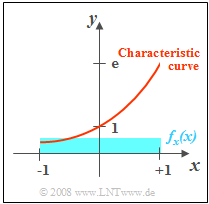# Exercise 4.2Z: Correlation between "x" and "e to the power of x"Given input PDF  $f_x(x)$  and characteristic curve  $y = {\rm e}^x$

Let the random variable  $x$  be uniformly distributed between  $-1$  and  $+1$.  Thus,

• the mean  $m_x = 0$,  and
• the variance  $\sigma_x^2 = 1/3$.

By the nonlinear characteristic  $y = g(x) = {\rm e}^x$  the random quantity  $y$  is formed.  Thus,  there is a fixed deterministic relationship between the two random variables  $x$  and  $y$.  The random variable  $y$  can only take values between  $1/{\rm e}$  and  ${\rm e}$.

For the probability density function,  one obtains for this range according to the principle  "Transformation of Random Variables":

$$f_y(y) = {\rm 1}/({\rm 2\it y}).$$

Hints:

$$y={\rm e}^{x}\approx 1+ \frac{ x}{1!} + \frac{{ x}^{\rm 2}}{\rm 2!}+ \frac{{x}^{\rm 3}}{\rm 3!}+ \frac{{x}^{\rm 4}}{\rm 4!}.$$

### Questions

1

What is the mean value  $m_y$  of the random variable  $y$?

 $m_y \ = \$

2

Calculate the standard deviation value  $\sigma_y$  of the random variable  $y$.

 $\sigma_y \ = \$

3

Which of the following statements are true regarding 2D–PDF  $f_{xy}(x, y)$?

 Outside the curve  $y = {\rm e}^x$:  ⇒   $f_{xy}(x, y)= 0$. For all two-dimensional values  $(x, {\rm e}^x)$  the PDF  $f_{xy}(x, y)$  is constant. The PDF describes a  "Dirac wall"  along the curve  $y = {\rm e}^x$. The height of the Dirac wall decreases from the lower left to the upper right.

4

Calculate the joint moment  $m_{xy}$  of the random variables  $x$  and  $y$,  that is,  the expected value of the product  $x \cdot y$.

 $m_{xy}\ = \$

5

Calculate the correlation coefficient  $\rho_{xy}$  between the random variables  $x$  and  $y$.  Interpret the result.

 $\rho_{xy}\ = \$

### Solution

#### Solution

(1)  The mean value  $m_y$  can be calculated in a known way from the PDF  $f_y(y)$.

• A second calculation possibility is based directly on the calculation rules for expected values:
$$m_y={\rm E}\big[ y\big] = \int_{-\infty}^{+\infty}g(x) \cdot f_x(x)\,\, {\rm d}x = {1}/{2}\cdot\int_{-1}^{1}{\rm e}^{ x}\,\,{\rm d}x=\rm {1}/{2}\cdot(e-e^{-1}) \hspace{0.15cm}\underline{= 1.175}.$$

(2)  For the second moment  (second order moment)  of the random variable  $y$  holds:

$$m_{2 y} = {\rm E}\big[ y^{\rm 2}\big] = {\rm E}[{\rm e}^{ 2 x}]= {1}/{2}\cdot\int_{-1}^{+1}{\rm e}^{2 x} \,\,{\rm d}x = {1}/{4}\cdot({\rm e}^{2}-{\rm e}^{-2}) = 1.813.$$
• From this we obtain by Steiner's theorem:
$$\sigma_y^{\rm 2} = m_{ 2 y}- m_{ y}^2 = {1}/{4}\cdot({\rm e}^{2}-{\rm e}^{-2})-{1}/{4}\cdot( {\rm e}^{2}-2+{\rm e}^{-2})={1}/{2}\cdot(1-{\rm e}^{-2})=0.432 \hspace{0.3cm} \Rightarrow \hspace{0.3cm}\sigma_y \hspace{0.15cm}\underline{= 0.658}.$$

(3)  Correct are  the proposed solutions 1, 3 and 4:

• Outside the curve  $y = {\rm e}^x$  the PDF is of course zero.
• Since the volume under the 2D–PDF is equal to  $1$,  the PDF values for the infinitely narrow region are infinite  $y = {\rm e}^x$.
• This means that the PDF describes a curved Dirac wall.
• Due to the decay of the PDF $f_y(y)$  with increasing  $y$,  the height of this Dirac wall decreases continuously from  $(-1, 1/{\rm e})$  to  $(+1, {\rm e})$  .

(4)  For the joint moment holds:

$$m_{xy} = {\rm E}\big[ x\cdot y \big] = {\rm E}\big[ x\cdot {\rm e}^{x} \big].$$
• With the series expansion given,  the approximation follows:
$$m_{xy} \approx {\rm E}\big[x\big] + {\rm E}\big[x^{\rm 2}\big] + \frac{1}{2} \cdot {\rm E}\big[ x^{\rm 3}\big] + \frac{1}{6} \cdot {\rm E}\big[ x^{\rm 4}\big]+ \frac{1}{24} \cdot {\rm E}\big[ x^{\rm 5}\big].$$
• Because of the symmetry of the random variable  $x$  holds for all odd values of  $k$:   $\rm E\big[\it x^{k}\rm \big] =\rm 0.$  Furthermore:
$${\rm E}\big[ x^{\rm 2}\big] = \sigma_{x}^{\rm 2}= \frac{1}{3}, \hspace{0.5cm} {\rm E}\big[ x^{\rm 4}\big] = \frac{1}{2}\int_{-1}^{+1} x^{\rm 4} \,\,{\rm d}x = \rm\frac{1}{5}\hspace{0.3cm} \Rightarrow \hspace{0.3cm}{\it m_{xy}} = \rm\frac{1}{3} + \frac{1}{6}\cdot\frac{1}{5} = \frac{11}{30}\hspace{0.15cm}\underline{\approx 0.367}.$$

(5)  Because of  $m_x = 0$  holds  $\mu_{xy} = m_{xy}$.  Thus,  for the correlation coefficient:

$$\it \rho_{xy} = \frac{\mu_{xy}}{\sigma_x \cdot \sigma_y}=\rm\frac{0.367}{0.577 \cdot 0.658}\hspace{0.15cm}\underline{ \approx 0.967}.$$
• Between  $x$  and  $y$  there is indeed a definite deterministic relation.
• But since there are also some nonlinear bindings in this,  the correlation coefficient  $\rho_{xy} \ne 1$.